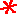Main      Site Guide### Scrambled Equations

• Scrambled Equations

In each of these puzzles, you are given a number that you must construct out of several other numbers. You do this by taking the numbers and performing addition, subtraction, multiplication, and/or division operations on them. Each number must be used in the calculations exactly once, and only these four operations listed may be used. You may parenthesize your expression however you wish. For example, 5 may be obtained from 1, 2, and 3, with the expression (3 + 2) × 1.

In many if not most cases, multiple solutions are possible, but usually only one is given on the solution page.

## #1

Make 42 out of 2, 3, 4, and 9.

Hint

Solution

## #2

Make 4 out of 2, 5, 6, and 8.

Hint

Solution

## #3

Make 40 out of 4, 6, 8, and 8.

Hint

Solution

## #4

Make 17 out of 1, 2, 6, and 7.

Hint

Solution

## #5

Make 31 out of 1, 2, 6, and 7.

Hint

Solution

## #6

Make 32 out of 1, 2, 6, and 7.

Hint

Solution

## #7

Make 33 out of 1, 2, 6, and 7.

Hint

Solution

## #8

Make 34 out of 1, 2, 6, and 7.

Hint

Solution

## #9

Make 43 out of 2, 5, 7, and 8.

Hint

Solution

## #10

Make 17 out of 1, 3, 3, and 6.

Hint

Solution

## #11

Make 80 out of 1, 3, 6, and 9.

Hint

Solution

## #12

Make 110 out of 1, 2, 7, and 8.

Hint

Solution

## #13

Make 29 out of 3, 4, 5, and 8.

Hint

Solution

## #14

Make 69 out of 1, 3, 9, and 9.

Hint

Solution

## #15

Make 35 out of 1, 2, 4, and 6.

Hint

Solution

## #16

Make 4 out of 1, 1, 6, and 7.

Hint

Solution

## #17

Make 1 out of 3, 3, 5, and 8.

Hint

Solution

## #18

Make 19 out of 2, 5, 8, and 9.

Hint

Solution

## #19Make 52 out of 2, 5, 8, and 9.

Hint

Solution

## #20

Make 27 out of 1, 6, 7, and 9.

Hint

Solution

## #21Make 68 out of 1, 2, 8, and 9.

Hint

Solution

## #22

Make 22 out of 1, 2, 2, and 6.

Hint

Solution

## #23

Make 23 out of 4, 4, 4, and 7.

Hint

Solution

## #24

Make 10 out of 4, 4, 4, and 9.

Hint

Solution

## #25

Make 23 out of 2, 6, 7, and 9.

Hint

Solution